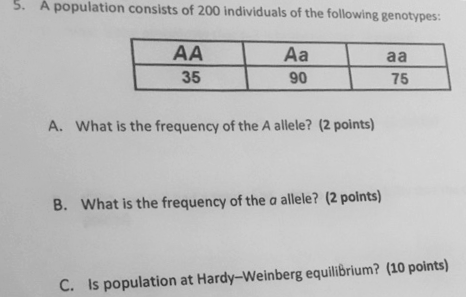# Problem: A population consists of 200 individuals with genotype information summarized below:A. What is the frequency of the A allele?B. What is the frequency of the a allele?C. Is population at Hardy-Weinberg equilibrium?

###### FREE Expert Solution

An allele frequency is can be computed as the total number of a particular allele for the gene divided by the total number of alleles for the gene in the given population.###### Problem Details

A population consists of 200 individuals with genotype information summarized below:

A. What is the frequency of the A allele?

B. What is the frequency of the a allele?

C. Is population at Hardy-Weinberg equilibrium?